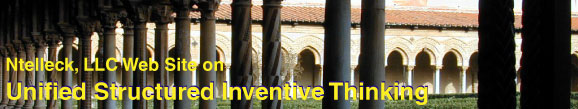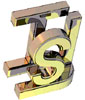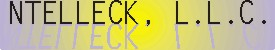PUZZLES========== SITE CONTENTS ========== Textbook Classes Publications & Essays eUSIT Ntelleck Services eUSIT eMail Registration USIT Q'a & A's Puzzles Resources Home Page

Puzzles and USIT

USIT-type solutions to puzzles are given here to illustrate how the insights of USIT can enable discovery of puzzle solutions. Of course, USIT is not required to solve puzzles. It is simply another tool in our toolbox of problem solving methodologies.

_____________________________________

P1. Ranking unequal weight billiard balls.

Given a balance and five billiard balls of unequal weight, rank them in order of decreasing weight using no more than seven measurements. [Given to me by a friend. The original source is unknown.]

P2. Somewhere on Earth, but Where?

A favorite puzzle of mine has an unusual presentation as the same situation posing two different problems. It goes as follows:

P2A: A hunter makes camp, then hikes three miles south, three miles east, and three miles north where he arrives at his starting point. In the process he shoots a bear. What color is the bear? Most adults get the answer to this problem quickly.

This problem has only one answer. When you get it, go on to the second problem.

P2B: This time the same problem situation is presented but with a different problem to be solved.

A hunter makes camp, then hikes three miles south, three miles east, and three miles north where he arrives at his starting point. Where did he start?

This problem has an infinite number of answers. In fact it has an infinite set of infinite number of solutions plus one, which of course is still an infinite number.

I would like to give proper attribution, but I’ve long forgotten the source of this puzzle. If you know its source, please let me know. I think I first heard it around 1969.

Pxx Do you have a puzzle to submit for this web site? If so, please send it to

Ntelleck@ic.net

_____________________________________

Hints to use if stumped

P1H. Ordering unequal weight billiard balls.

Notice that the type of ball should have no bearing on the problem; if the puzzle works for one type of ball it should work for another. Hence, eliminate "billiard". Why should "ball" be significant? Attributes of ball that come to my mind are first shape (spherical) and possibly second would be elasticity. Since this is a weighing problem, neither shape nor elasticity are relevant attributes. Hence, eliminate "ball" and substitute the generic word "object". Ordering by decreasing weight is only the reverse of ordering by increasing weight, if you get one you have the other. If no approach seems to make any headway, simplify the problem by starting with a smaller number of objects. Once you've minimized the number of steps to a solution, you can see your way to tackling a larger number of objects. The puzzle might now be stated as, "Rank five unequal weight objects in seven measurements with a balance."

P2H Somewhere on Earth, but Where?

The second problem has nothing to do with bears, camps or hunters. It is a simple problem in spherical geometry that you can solve in your head without equations. But, if it doesn’t happen quickly enough, an answer aided by USIT is given below.

Puzzle USIT-Solutions

Click on the solutions you wish to see.

P1S. Rankring unequal weight billiard balls

---------------------------------------